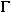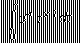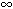DOC HOME SITE MAP MAN PAGES GNU INFO SEARCH PRINT BOOK

# gamma(3M)

gamma, lgamma, tgamma -- gamma functions

## Synopsis

```   cc [flag ...] file ...  -lm [library ...]

#include <math.h>

double gamma(double x);

float gammaf(float x);

long double gammal(long double x);

double lgamma(double x);

float lgammaf(float x);

long double lgammal(long double x);

extern int signgam;

double tgamma(double x);

float tgammaf(float x);

long double tgammal(long double x);
```

## Description

gamma, gammaf, gammal, lgamma, lgammaf and lgammal return the natural logarithm of the gamma value of x. Where(x) is defined as:The sign of(x) is returned in the external integer signgam. The argument x must be positive and greater than zero. A range error occurs if x is too large.

tgamma, tgammaf and tgammal return the gamma value of x. The argument x must be positive and greater than zero. A range error may occur if the magnitude of x is too large or too small.

### Errors

If x is ±, ±0 or a negative integer, gamma, gammaf, gammal, lgamma, lgammaf and lgammal return +HUGE_VAL, +HUGE_VALF or +HUGE_VALL (respectively) and errno is set to ERANGE.

If x is -, ±0 or a negative integer, tgamma, tgammaf and tgammal return IEEE NaN on systems that support it and raise the invalid operation exception. Otherwise, they return 0. errno is set to EDOM.

If x is +tgamma, tgammaf and tgammal return +HUGE_VAL, +HUGE_VALF or +HUGE_VALL and errno is set to ERANGE.

If the correct value would overflow, all gamma functions return a value that compares equal to +HUGE_VAL, +HUGE_VALF or +HUGE_VALL and set errno to ERANGE.

On systems that support IEEE NaN, if x is a quiet NaN, that value is returned. If x is a signaling NaN, a quiet NaN is returned and the invalid operation exception is raised. In either case, errno is set to EDOM.

If the program was compiled with the -Xt compilation mode, a value that will compare equal to HUGE is returned instead of HUGE_VAL. In addition, for non-positive integer arguments, a message indicating SING error is printed on the standard error output. These error handling procedures may be changed with the function matherr.

## References

cc(1), exp(3M), matherr(3M), values(5)